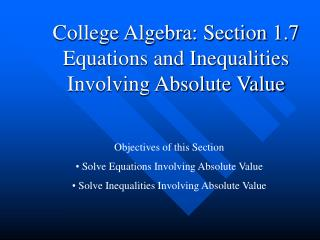# College Algebra: Section 1.7 Equations and Inequalities Involving Absolute Value - PowerPoint PPT PresentationDownload PresentationCollege Algebra: Section 1.7 Equations and Inequalities Involving Absolute Value

College Algebra: Section 1.7 Equations and Inequalities Involving Absolute ValueDownload Presentation## College Algebra: Section 1.7 Equations and Inequalities Involving Absolute Value

- - - - - - - - - - - - - - - - - - - - - - - - - - - E N D - - - - - - - - - - - - - - - - - - - - - - - - - - -
##### Presentation Transcript

1. College Algebra: Section 1.7Equations and Inequalities Involving Absolute Value • Objectives of this Section • Solve Equations Involving Absolute Value • Solve Inequalities Involving Absolute Value

2. Equations Involving Absolute Value If the absolute value of an expression equals some positive number a, then the expression itself equals either a or -a. Thus,

3. Theorem

4. Ex. Solve y + 21 ≥ 7 • Draw the “river” • Subtract 21 from both sides • Simplify • Check your answer • Graph the solution - 21 -21 y ≥ -14 (-14) + 21 ≥ 7 7 ≥ 7 ● -15 -14 -13

5. Ex. Solve 8y + 3 > 9y - 14 - 8y - 8y 3 > y - 14 + 14 + 14 17 > y y < 17 8(16) + 3 > 9(16) – 14 131 > 130 • Draw “the river” • Subtract 8y from both sides • Simplify • Add 14 to both sides • Simplify • Rewrite inequality with the variable first • Check your answer • Graph the solution o 16 17 18

6. Ex. Solve 8y + 3 > 9y - 14 - 8y - 8y 3 > y - 14 + 14 + 14 17 > y y < 17 8(16) + 3 = 9(16) – 14 131 > 130 Big Tip!!! At the end of solving inequality, always put the variable at the LEFT hand side. Then arrow of the inequality sign tells you the correct graph y < 17 The graph should toward to the left. o 16 17 18

7. To Solve the Absolute Value Inequalities • 1. Isolate the absolute value expression. • 2. Make sure the absolute value inequality can be defined. • 3. For any defined absolute value inequality with template: • |X|  a, where a > 0 and  = <, >, ≤, ≥ • write the corresponding compound inequalities by following the • rules: • a) if “|X| > a” or “|X| ≥ a”, meaning “greator”, “leaving the jail” • b) set “jail boundaries” as “–a” and “a” • c) write compound inequalities as • X < –aor X > a meaning “stay left to the left • boundary or right to the right boundary” • d) if “|X| < a” or “|X| ≤ a”, meaning “less thand”, “going • to the jail” • e) set “jail boundaries” as “–a” and “a” • f) write compound inequalities as • –a < X < a (and) meaning “stay between the two • boundaries” • 4. Solve the converted compound inequalities.

8. Absolute Value Inequality Solve | x – 3 | < 5 • The absolute value expression is isolated • It is a well defined absolute value inequality • It is a “less thand” inequality. (go to the jail) • set jail boundaries: –5, and 5 • write compound inequalities: (stay in between the jail boundaries) • –5 < x – 3 < 5 • –2 < x < 8 +3 +3 +3

9. Absolute Value Inequality You try this! Solve | x + 4 | < 1 • The absolute value expression is isolated • It is a well defined absolute value inequality • It is a “less thand” inequality. (go to the jail) • set jail boundaries: –1, and 1 • write compound inequalities: (stay in between the jail boundaries) • –1 < x + 4 < 1 • –5 < x < –3 – 4 – 4 – 4

10. Absolute Value Inequality Solve | 2x + 3 | – 3 ≥ 2 • The absolute value expression is NOT isolated • | 2x + 3 | – 3 ≥ 2 • | 2x + 3 | ≥ 5 • It is a well defined absolute value inequality • It is a “greator” inequality. (leaving the jail) • set jail boundaries: –5, and 5 • write compound inequalities: (stay left to left boundary and right to the right boundary) • 2x + 3 ≤ –5 or 2x + 3 ≥ 5 • 2x ≤ –8 or 2x ≥ 2 • x ≤ –4 orx ≥ 1 + 3 + 3 – 3 – 3 – 3 – 3

11. Absolute Value Inequality You try this! Solve | 4x – 3 | + 5 ≥ 8 • The absolute value expression is NOT isolated • | 4x – 3| + 5 ≥ 8 • | 4x – 3 ≥ 3 • It is a well defined absolute value inequality • It is a “greator” inequality. (leaving the jail) • set jail boundaries: –3, and 3 • write compound inequalities: (stay left to left boundary and right to the right boundary) • 4x – 3 ≤ –3 or 4x – 3 ≥ 3 • 4x ≤ 0 or 4x ≥ 6 • x ≤ 0 orx ≥ 3/2 – 5 – 5 + 3 + 3 + 3 + 3

12. Summary • Before solving absolute value equations or inequalities, you MUST isolate the absolute value expression and check them are defined or not. • Remember telling yourself the jail story. It will help you set up the correct equations or compound inequalities. • Solve the equations or compound inequalities and don’t forget the learned knowledge such as when multiplying or dividing a negative number, you need flip the inequality sign.

13. Solution Set:

14. Theorem